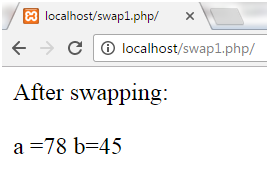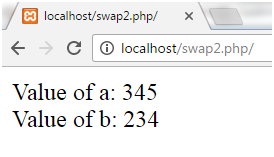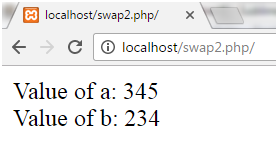Q:

# Swapping two numbers in Php

Two numbers can be swapped or interchanged. It means first number will become second and second number will become first.

For example

a = 20, b = 30

After swapping,

a = 30, b = 20

There are two methods for swapping:

• By using third variable.
• Without using third variable.

## Swapping Using Third Variable

Swap two numbers 45 and 78 using a third variable.

Example:

``````<?php
\$a = 45;
\$b = 78;
// Swapping Logic
\$third = \$a;
\$a = \$b;
\$b = \$third;
echo "After swapping:<br><br>";
echo "a =".\$a."  b=".\$b;
?>  ``````

Output:## Swapping Without using Third Variable

Swap two numbers without using a third variable is done in two ways:

• Using arithmetic operation + and ?
• Using arithmetic operation * and /

Example for (+ and -):

``````<?php
\$a=234;
\$b=345;
//using arithmetic operation
\$a=\$a+\$b;
\$b=\$a-\$b;
\$a=\$a-\$b;
echo "Value of a: \$a</br>";
echo "Value of b: \$b</br>";
?>  ``````

Output:Example for (* and /):

``````<?php
\$a=234;
\$b=345;
// using arithmetic operation
\$a=\$a*\$b;
\$b=\$a/\$b;
\$a=\$a/\$b;
echo "Value of a: \$a</br>";
echo "Value of b: \$b</br>";
?>  ``````

Output: